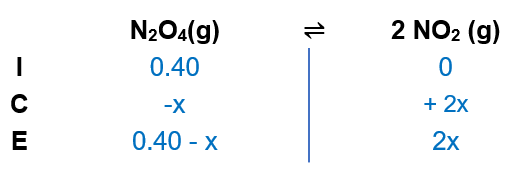# Problem: For the reaction N2O4(g) ⇌ 2NO2(g), Kp = 0.25 at a certain temperature. If 0.040 atm of N2O4 is reacted initially, calculate the equilibrium partial pressures of NO 2(g) and N2O4(g).

###### FREE Expert Solution

(1) Setup an ICE chart(2) Calculating for the concentration of N2O4 and NO2

82% (86 ratings)###### Problem Details

For the reaction N2O4(g) ⇌ 2NO2(g), Kp = 0.25 at a certain temperature. If 0.040 atm of N2O4 is reacted initially, calculate the equilibrium partial pressures of NO 2(g) and N2O4(g).